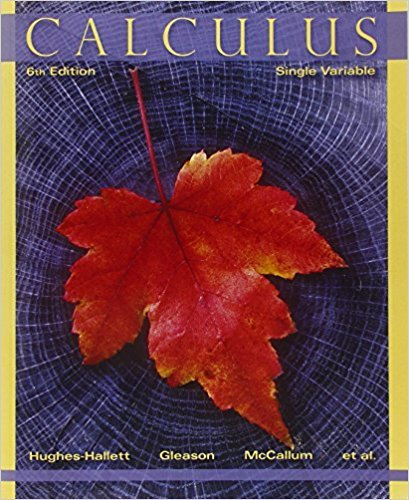×
×

# Solutions for Chapter 8.2: APPLICATIONS TO GEOMETRY## Full solutions for Calculus: Single Variable | 6th Edition

ISBN: 9780470888643Solutions for Chapter 8.2: APPLICATIONS TO GEOMETRY

Solutions for Chapter 8.2
4 5 0 416 Reviews
14
1
##### ISBN: 9780470888643

This textbook survival guide was created for the textbook: Calculus: Single Variable , edition: 6. Chapter 8.2: APPLICATIONS TO GEOMETRY includes 81 full step-by-step solutions. This expansive textbook survival guide covers the following chapters and their solutions. Calculus: Single Variable was written by and is associated to the ISBN: 9780470888643. Since 81 problems in chapter 8.2: APPLICATIONS TO GEOMETRY have been answered, more than 33620 students have viewed full step-by-step solutions from this chapter.

Key Calculus Terms and definitions covered in this textbook

P(A or B) = P(A) + P(B) - P(A and B). If A and B are mutually exclusive events, then P(A or B) = P(A) + P(B)

• Bar chart

A rectangular graphical display of categorical data.

• Blocking

A feature of some experimental designs that controls for potential differences between subject groups by applying treatments randomly within homogeneous blocks of subjects

• Components of a vector

See Component form of a vector.

• equation of a quadratic function

ƒ(x) = ax 2 + bx + c(a ? 0)

• Instantaneous rate of change

See Derivative at x = a.

• Inverse composition rule

The composition of a one-toone function with its inverse results in the identity function.

• Inverse cosecant function

The function y = csc-1 x

• Irrational numbers

Real numbers that are not rational, p. 2.

• Linear inequality in x

An inequality that can be written in the form ax + b < 0 ,ax + b … 0 , ax + b > 0, or ax + b Ú 0, where a and b are real numbers and a Z 0

• Midpoint (on a number line)

For the line segment with endpoints a and b, a + b2

• Negative angle

Angle generated by clockwise rotation.

• Paraboloid of revolution

A surface generated by rotating a parabola about its line of symmetry.

• Pie chart

See Circle graph.

• Rational zeros

Zeros of a function that are rational numbers.

• Reciprocal identity

An identity that equates a trigonometric function with the reciprocal of another trigonometricfunction.

• Reference angle

See Reference triangle

• Vertices of a hyperbola

The points where a hyperbola intersects the line containing its foci.

• Weights

See Weighted mean.

• y-intercept

A point that lies on both the graph and the y-axis.

×